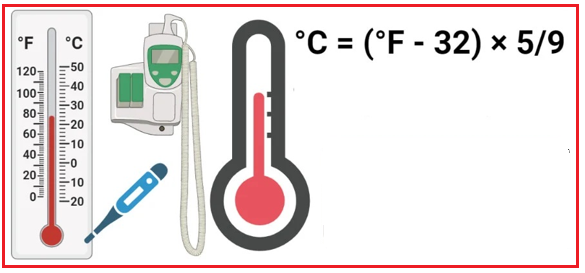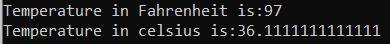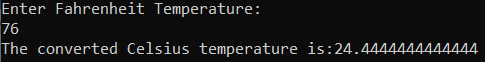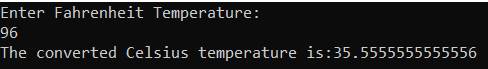# Fahrenheit to Celsius in C#

## Fahrenheit to Celsius in C# with Examples

In this article, I am going to discuss how to convert Fahrenheit to Celsius in C# with Examples. Please read our previous article, where we discussed Celsius to Fahrenheit in C#.

##### How to Convert Fahrenheit to Celsius in C#?

Celsius scale is a temperature scale based on the freezing point of water at 0-degree centigrade and the boiling point of water at 100-degree centigrade. Fahrenheit scale is a temperature scale that is based on the freezing point of water at 32-degree Fahrenheit and the boiling point of water at 212-degree Fahrenheit.

The formula of Fahrenheit to Celsius is C= (F – 32) * 5/9, where, F is degree Fahrenheit and C is Celsius Fahrenheit. For better understanding, please have a look at the below image.##### Example:

The formula of Fahrenheit to Celsius is: C= (F – 32) * 5/9= 36.11

In this program, we use going to use the following data type

1. Float: Floating-point numbers are used for decimal and exponential values. It is a primitive data type. It has 6 decimal digits of precision. Example: float volume = 20.93333
2. Double: Double is also a data type that is used to represent the floating-point numbers. It has 15 decimal digits of precision. Example: double y = 20.9333333333333
##### Logic to convert Fahrenheit to Celsius in C#

To convert Fahrenheit to Celsius, we need to follow the below steps.

1. We will define Celsius and Fahrenheit.
2. Then we will assign the value of Fahrenheit.
3. Then we will calculate Celsius by using the formula: C= (F-32) * 5/9
4. And the resulting output will store in Celsius.
##### Example: Convert Fahrenheit to Celsius in C#

The following C# Program will Convert Fahrenheit to Celsius.

```using System;
class FahrenheitToCelsius
{
static void Main(string[] args)
{
double Fahrenheit = 97;
Console.WriteLine("Temperature in Fahrenheit is:" + Fahrenheit);
double Celsius = (Fahrenheit - 32) * 5 / 9;
Console.WriteLine("Temperature in celsius is:" + Celsius);
}
}
```
###### Output:##### Example: Taking input from the user

In the following C# program, we will take the Fahrenheit from the user and then calculate the Celsius and print it on the console.

```using System;
class FahrenheitToCelsius
{
static void Main(string[] args)
{
Console.WriteLine("Enter Fahrenheit Temperature:" );
double Celsius = (Fahrenheit - 32) * 5 / 9;
Console.WriteLine("The converted Celsius temperature is:" + Celsius);
}
}
```
###### Output:##### C# Code (Using function)

In the below example, we have created one method to calculate the Celsius from Fahrenheit.

```using System;
class FahrenheitToCelsius
{
static void Main(string[] args)
{
Console.WriteLine("Enter Fahrenheit Temperature:");
double Celsius = FahrenheitCelsius(Fahrenheit);
Console.WriteLine("The converted Celsius temperature is:" + Celsius);